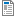National Curriculum

# Year 2/3 Maths Plans

Hamilton provide Mixed Year 2/3 weekly maths plans (below). We hope, in time, to develop maths blocks for this mixed year combination. Find out more about our plans to phase out these mixed age plans in favour of Year 2/3 maths blocks which we believe offer all the advantages of the weekly plans and more.

Supporting documents for set
• Week
• Title
1
+ Details
Number and place value

Year 2: Place 2-digit numbers on a line. Round 2-digit numbers; Order and compare 3-digit numbers. Understand place value additions.  Year 3: Partition and represent 3-digit numbers using PV cards and multi-base equipment (Dienes). Place 3-digit numbers on an empty number line; Order, compare and find a number between 3-digit numbers. Round 3-digit numbers to the nearest 10 and 100. Count past 1000 and begin to understand place value in 4-digit numbers.Summer Week 1 Plan
•Summer Week 1 Image Resource
•ITP Place Value
2
+ Details

Year 2: Double and halve using partitioning. Add 2-digit numbers by partitioning or counting on. Subtract 2-digit numbers counting back. Year 3: Use expanded and compact addition to add any pair of 3-digit numbers. Use rounding to estimate totals. Find patterns and make generalisations. Use Frog to subtract 2-digit numbers from 3-digit numbers, e.g. 137 – 72 and then numbers within the same century, e.g. 472 – 427.Summer Week 2 Plan
•Summer Week 2 Image Resource
•ITP Place Value
3
+ Details
Multiplication and division

Year 2: Multiply and divide by 2, 5 and 10 using beaded lines. Understand multiplication as the inverse of division. Year 3: Double numbers to 50 and halve numbers to 100 using partitioning. Know times tables and division facts (1x, 2x, 3x, 4x, 5x, 8x, 10x). Begin to use the grid method to multiply 2-digit numbers (<30) by 1-digit numbers. Find and test rules.

4
+ Details
Shape and time

Year 2: Identify 3D shapes and their properties. Tell the time to nearest quarter of an hour and to five-minute intervals.  Year 3: Describe, name and sort 3D shapes, learn and use correct vocabulary; count faces, vertices and edges. Begin to calculate time intervals in hours and minutes.

5
+ Details

Year 2: Subtract by counting up (answers less than 20) and by counting back. Choose whether to count back or up to subtract. Year 3: Add three or four 2-digit numbers using expanded then compact written addition. Find and test rules. Find change from £5, £10 and £20 and find differences between amounts of money (using Frog). Includes bar model examples.

6
+ Details
Number, sequences and fractions

Year 2: Count in 2s, 5s, 10s and 3s. Recognise multiples of 2, 5, 10 and 3. Find ½s, ¼s, ¾s and 1/3 of amounts.  Year 3: Count in steps of 50 and 100. Count in steps of 4 and 8. Work out the rule for a sequence. Understand tenths, and find tenths of amounts. Understand fractions as both numbers and operators. Find unit fractions of amounts. Includes bar model examples.

7
+ Details
Mental multiplication and division

Year 2 objectives: Half or double a 2-digit number; Know that multiplication is the inverse of division; Multiply and divide using beaded or landmarked lines. Year 3 objectives: Scale up by multiplying by 4 (double twice) and by 10; Scale down by dividing by 4 (halve twice) and by 10; Divide numbers just beyond the times tables (no remainders); Divide numbers just beyond the times tables (with remainders).

8
+ Details
Measures and data

Year 2: Estimate, measure and compare capacities and weights, choosing and using suitable non-standard and standard units and suitable measuring instruments. Answer a question by collecting & recording data, and representing it as block graphs and pictograms to show results.  Year 3: Measure in litres and millilitres. Convert between whole/half litres and millilitres. Measure/convert weight in kg and g. Estimate, measure objects and record in tables. Represent and interpret data in bar charts; Measure in m, cm and mm. Draw a bar chart where one square represents 10 units. Measure perimeters of 2D shapes.

9
+ Details

Year 2 objectives: Add pairs of 2-digit numbers by partitioning or counting on; Subtract by counting up or counting back; Choose to add or subtract to solve money word problems. Year 3 objectives: Add three or four 2-digit numbers using compact addition; Estimate answers; Use column addition to add three 3-digit numbers; Use column addition to add two amounts of money; Use counting up (Frog) to find change from £5, £10, £20 and £100. Includes bar model examples.

10
+ Details
Fractions and time

Year 2: Find halves, thirds and quarters of amounts. Count in fractions. Tell the time in analogue and digital to the nearest 5 minutes.  Year 3: Find unit and non-unit fractions of amounts. Find fractions equivalent to ½ and ¼. Add and subtract fractions with the same denominator within one whole. Tell the time to nearest minute. Compare time durations. Includes bar model examples.

11
+ Details
Addition and subtraction, multiplication and division

Year 2 objectives: Use and recognise coins; Add 2-digit amounts using partitioning or counting up; Subtract by finding a difference or counting back; Use addition and subtraction to solve 2-step money problems. Year 3 objectives: Add 3-digit numbers using place value; Add near multiples of 100; Subtract 3-digit numbers using place value; Subtract near multiples of 100; Use the grid method to multiply 2-digit numbers (numbers < 40) by 1-digit numbers; Divide numbers within and just beyond the times tables (with remainders); Solve correspondence problems.